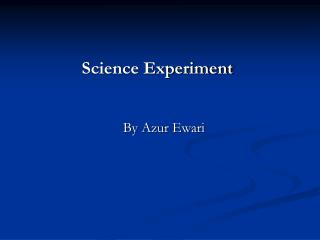DownloadDownload PresentationScience Experiment

# Science Experiment

Télécharger la présentation## Science Experiment

- - - - - - - - - - - - - - - - - - - - - - - - - - - E N D - - - - - - - - - - - - - - - - - - - - - - - - - - -
##### Presentation Transcript

1. Science Experiment By Azur Ewari

2. Big Question How long does it take different volumes of water to freeze at this temprature? This experiment is to see if a bigger amount of water freezes faster than a smaller volume of water.

3. Hypothesis My hypothesis is that if you have a bigger volume of water it will take longer time for the molecules to go farther apart and make the crystals then it will take longer time for it to freeze into solid ice, and if you had a less volume of water it would take fewer time for it to form crystals and become solid ice.

4. Interesting facts • When water freezes that is the point when the kinetic energy of water is low enough that allows water to change to solid state of matter. • As the water gets colder, its molecules slow down and begin moving far apart. • Kinetic energy is the energy possessed by a body because of its motion, equal to one half the mass of the body times the square of its speed. • It takes a temperature of -5 degrees Celsius (this is just an example, not an actual value) for the water to break its association with the ions and freeze as a pure substance. • You'll find that adding solute to water generally lowers its freezing point which is why salt melts ice.

5. Materials • measuring cup • Two containers • Pitcher with water • Thermometer • freezer

6. Procedure • I will fill the measuring cup to 4 ounces. • Then I will pour the water into the first cup. • I will then fill the measuring cup to 8 ounces. • Then I’ll pour it into the second cup. • Then I’ll take a picture of both of them now. • After that I will put them both into the freezer or fridge and set for around 32 degrees F. • Every ½ an hour then when ice crystals form 5to10min. • When I do this I will also see which one turns to ice first. • Once one has turned to ice I’ll take a picture of both and show whether more water takes longer or smaller water takes longer to freeze.

7. Control/Variables • My control is that during the experiment I used the same freezer. • The variables are the different volumes that I used and they were 4oz and 8oz.

8. Observations • One observation I made is that the 4oz cooled faster than the 8oz. • Another observation that I made is that once they got started they slowed down a bit. • I also noticed that the 4oz got ice crystals faster than the 8oz did. • Also, I observed that after the 4oz started to slow the 8oz started to gain.

9. Data

10. Analysis of data My data is accurate but I was off because my freezer was set for 20 degrees instead of 32 degrees. I think my numbers would have changed if it was at 32 degrees.

11. Conclusion • My Hypothesis was correct because the lesser volume froze first. • What I would change in the experiment is change the temperature from 20 to 32 degrees. • A new question that I have is what is the effect of other liquids at a certain temperature.

12. References http://www.answerbag.com/q_view/111892 (September 2009)http://wiki.answers.com/Q/Why_does_water_freeze_at_32_degrees_Fahrenheit (September 2009)http://www.ehow.com/how-does_4600114_water-freeze.html (September 2009)Day.T (2007) WaterTaylor.B (2008) Understanding Landformes